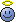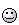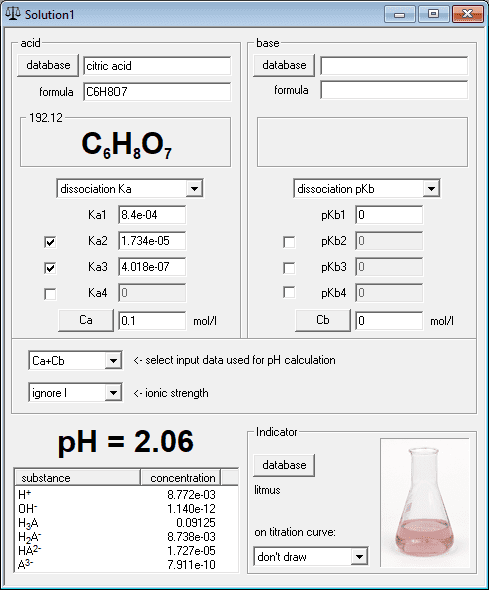# Estimating pH Without [H+][A-]: Is There Another Way?

• Biology
• TytoAlba95
In summary, the pH of a concentrated solution of weak acid is approximately -2log3 + 3log10 (rounded off 9.1 into 9).f

#### TytoAlba95

Homework Statement
The acidic nature of orange juice is mainly due to citric acid. What would be the pH of 0.1M citric acid, if Ka is 8.4 x 10^-4?
Relevant Equations
pH= pKa + log ([H+][A-]/[HA])
How can we estimate the pH when the [H+][A-] is not provided. Is there some other formula?

Are you using all the relevant information you have, even if you are not retold it when the question is asked?
Electroneutrality?
It also helps if you know that a concentrated solution of weak acid will be little dissociated which allows an easy approximation though if you have any qualms about that you can still calculate it more accurately as a check.

Last edited:
The equation you wrote is wrong (check the part under log) and - assuming you meant Henderson-Hasselbalch equation - not applicable to this question.

This is just about a solution of a weak acid, not about a buffer solution.

•epenguin
The equation you wrote is wrong (check the part under log) and - assuming you meant Henderson-Hasselbalch equation - not applicable to this question.

This is just about a solution of a weak acid, not about a buffer solution.

Thank you for the pointer.
pH= pKa + log ([H+][A-]/[HA]) -> pH= pKa + log ([A-]/[HA]) .

New attempt:

Ka= x2 / C-x => 10-6 = x2 / 0.01-x
=> x2 + 10-6 - 10-8 = 0

Ka is not 10-6, C is not 0.01. Not only you started with wrong values, you also made several simple math mistakes while trying to solve.

At least it looks like you are trying to start from a correct equation this time. I wonder if you know what x is and where does this equation come from.

Yes the first equation of your new attempt looks as if it could be right if you used the proper brackets. And then the right numerical values as Borek says.
Alternatively or additionally even your corrected equation before that (which is now the Henderson-Hasselbalch equation) can be used together with the information I mentioned previously.
You seem to have used one of my suggestions, not the other.

Start from first principles.
What is the equilibrium expression for

HA <——> H+ + A-

Last edited:
You seem to have used one of my suggestions, not the other.

It also helps if you know that a concentrated solution of weak acid will be little dissociated which allows an easy approximation though if you have any qualms about that you can still calculate it more accurately as a check.

You meant, excluding the subtraction of x from C in the denominator, right?

Ka is not 10-6, C is not 0.01. Not only you started with wrong values, you also made several simple math mistakes while trying to solve.

At least it looks like you are trying to start from a correct equation this time. I wonder if you know what x is and where does this equation come from.

Oh! I'm sorry. I hurriedly solved the sum, which is why it was full of mistakes.
Ka= x2 / C
=> 8.4 x 10-4 = x2 / 0.01
=> 8.4 x 10-6 = x2
=> 2.8 x 10 -3= x
I think this time I nailed it!

Yes that was what I meant.
But you are not finished.
Everyone makes a % of mistakes in arithmetic etc. or can mix one figure with another, I am never that confident I haven't,, but your mistake rate is at alarm level!You have now made the citric acid concentration 0.01 M, wasn't it 0.1 M?
And you haven't answered the question which was what is the pH?! So you give your evil examiners every excuse to fail you!

If you complete the answer I have a couple of other things to tell you.

Last edited:
Ka= x2 / C-x

Ka= x2 / C

Either - either. These are two different equations. Each has its applicability, but you don't use them at random, you need to check which one can be used.

Ka= x2 / C
=> 8.4 x 10-4 = x2 / 0.01
=> 8.4 x 10-6 = x2
=> 2.8 x 10 -3= x
I think this time I nailed it!

I'm sorry, for making such silly mistakes again. Doing maths on computer is really not my thing.

Ka= x2 / C
=> 8.4 x 10-4 = x2 / 0.1
=> 8.4 x 10-5 = x2
=> 9.1 x 10 -3= x
Therefor pH= -log [H] = -log(9.1 x 10 -3)
= -2log3 + 3log10 (rounded off 9.1 into 9)
= -2x 0.477 + 3
= 2.046

@Borek
These are two different equations. Each has its applicability, but you don't use them at random, you need to check which one can be used.
Yup. By now I know where to use them. Thanks for the pointer.

@epenguin
If you complete the answer I have a couple of other things to tell you.

Last edited:
= 2.046

@epenguin

It was just to say that from the HH equation in #4, electroneutrality condition [A-] = [H+], definition of pH, and approximation (C - [H+]) ≈ C you can get the equation, often quoted

pH ≈ (pK - log C)/2

This is completely equivalent to what you did. From that I get pH = 2.033 but the small difference is likely due to your rounding.

It's also convenient for quick mental calculation, useful in laboratory thinking. The pH of a 1M solution of weak acid equals half the pKa; then for every 10 fold dilution it increases by 0.5 (for as long as the approximation you made holds good, which it doesn't very by the time the concentration gets to the order of magnitude of Ka).

The other thing is that citric acid has three acid dissociations from its three carboxylic groups, in your solution only one of these is significantly dissociated, so you don't have to worry here about the others whose pK's are about 4.76 and 6.4

Last edited:
•TytoAlba95(pKa2 = 4.761, pKa3 = 6.396, by default I am using a bit different value for Ka1, but modified it here to the value from the initial post)

•TytoAlba95